# Multiplying Decimals by 10

Alignments to Content Standards: 5.NBT.A.2

1. Explain why $0.4\times10=4$.
2. Explain why $3.4\times10=34$.

Draw pictures to illustrate your explanations.

## IM Commentary

The purpose of this task is to help students understand and explain why multiplying a decimal number by 10 shifts all the digits one place to the left. This understanding builds on work around place-value that students have done with whole numbers in 3rd and 4th grade (see especially 3.NBT.3 and 4.NBT.1). This is an instructional task that the teacher can give students before discussing this "digit shifting" principle for multiplying decimal numbers by 10.

The task begins with a decimal number with only one non-zero digit so that students can see how to it works in the simplest case. Note that the seeing that 10 groups of (4 groups of 0.1) is equal to 4 groups of (10 groups of 0.1) requires both the commutative and associative properties of multiplication:

\begin{align} 10\times0.4 &=10\times(4\times 0.1)\\ &=(10\times4)\times 0.1 \\ &=(4\times10)\times 0.1 \\ &= 4\times(10\times 0.1) \end{align}

However, this equivalence is much easier to see with a simple diagram. The pictures shown in the solution are meant to reflect how one could use base-ten blocks to show these relationships; sometimes it helps to put the groups of 10 together oneself and replace them with the next larger power of 10. On the other hand, students will be using the "ten-stick" to represent 1 (so that the small cubes can represent one-tenth of it), so if the teacher wishes to supply base-ten blocks, it might be helpful to suggest this use ahead of time. The picture at the top of the solution shows what each of the pieces represent to correspond to the solutions given.

Using bundles of popsicle sticks, straws, or toothpicks works even better, since bundled objects give students the opportunity to bundle the groups of ten for themselves. They key is to realize that when you have 10 times a base-ten number, you have 10 copies of every base-ten unit that comprises the number, and each of these can be bundled to make the next larger unit.

## Solution1. 0.4 is 4 tenths.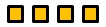$0.4\times10 = 10\times0.4$ is 10 groups of 0.4, which we can think of as 10 groups with 4 tenths in each group.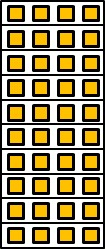We can think of this, in turn, as 4 groups with 10 tenths in each group.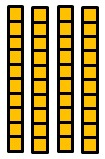Since 10 tenths is 1 one, we can think of this as 4 ones.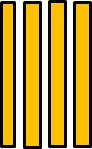So $0.4\times10 =4$.

2. 3.4 is 3 ones and 4 tenths.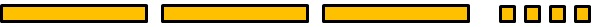$3.4\times10 = 10\times3.4$ is 10 groups of 3.4, which we can think of as 10 groups with 3 ones and 4 tenths in each group.We can think of this, in turn, as 3 groups with 10 ones in each group and 4 groups with 10 tenths in each group.Since 10 ones is 1 ten and 10 tenths is 1 one, we can think of this as 3 tens 4 ones.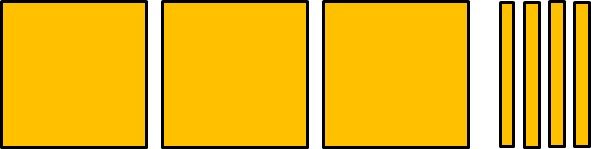So $3.4\times10 =34$.